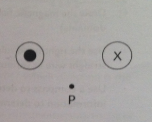# Problem: Two wires lie perpendicular to the plane of the paper, and equal electric currents pass through the paper in the directions shown. Point P is equidistant from the two wires.Construct a vector diagram showing the direction of the resultant magnetic field at point P due to currents in these two wires. Explain your reasoning,

###### FREE Expert Solution
84% (224 ratings)###### Problem DetailsTwo wires lie perpendicular to the plane of the paper, and equal electric currents pass through the paper in the directions shown. Point P is equidistant from the two wires.

Construct a vector diagram showing the direction of the resultant magnetic field at point P due to currents in these two wires. Explain your reasoning,